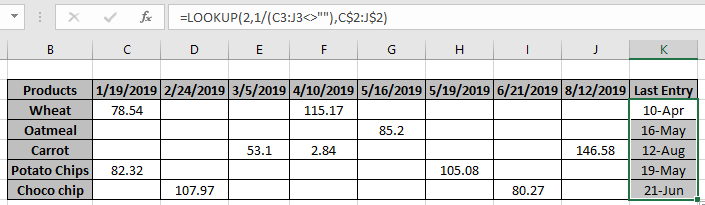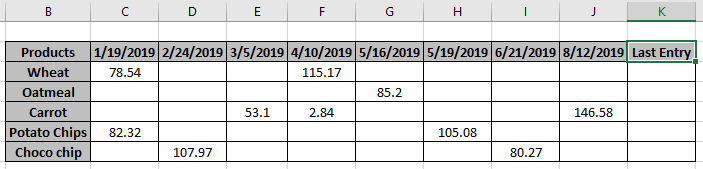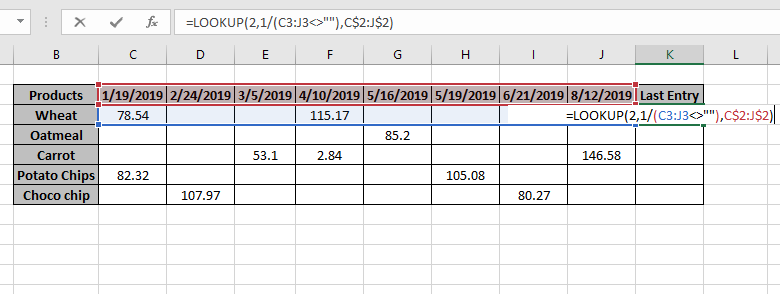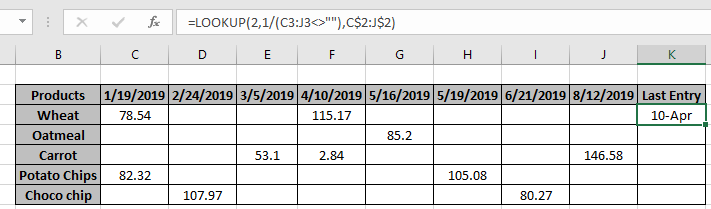# LOOKUP date with last value in listIn this article, we will learn how to LOOKUP date with last entry in a row or column in Excel.

Scenario:

We have a long table of entries of products with blank cells between the values. We need to find the last entry of the product ordered and date associated with it.

How to solve the problem?

We will be using the LOOKUP function. Let's first get some knowledge about the function. LOOKUP function finds the estimated value in the range and returns the value from the range or its corresponding value.

Syntax:

= LOOKUP ( lookup_value, lookup_vector, [result_vector] )

lookup_value: value to look up for.
lookup_vector: array where the function looks for the lookup_value.
[result vector]:[optional] if the return array is different from the lookup array.

But here we will be using one of the attributes of the LOOKUP function i.e. if LOOKUP function can't find an exact match, it will match with just the previous value when it stops. For this, we will be using the below syntax.

Generic Formula:

= LOOKUP ( 2 , 1 / ( list<>"" ) , date_range )

list : lookup list
date_range : date value to be returned from the range

Example:

Here we have products delivered with their date and amount and we need to find the last delivered product date for each product.So here we have some blank cells between the date value entries.
Formula:

= LOOKUP ( 2 , 1 / ( C3 : J3 <>"") , C\$2:J\$2 )

C\$2:J\$2 : fixed date_range

Explanation:

• <>"" this pattern with a list returns an array of TRUEs and FALSEs. TRUE value corresponding to existing values and FALSE values corresponding to blank cells.
• TRUE values are considered as a numerical value 1 and FALSE values as 0.
• LOOKUP function looks up for the numerical value 2 in an array of 1s and 0s.
• So when the function doesn't find the value 2. So it looks backward in the array for the least closest value to 2 which is 1 here.
• The function returns the date related to the last entry.Press Enter to get the results.As you can see, 10-Apr is the last entry date for the Wheat product.

Now copy the formula to other cells using the shortcut Ctrl + D or drag down cell option from right bottom of the cell.As you can see, the function returns the value as required. You can customize Excel functions as required.

Here we have observational notes for using the formula.

Notes:

1. The formula returns #NA error if no match is found in the array.
2. The formula checks the lookup value first and then looks up for the just previous value, if the lookup value is not found.
3. All excel formula always returns the first value found in the table array.
4. The above explained formula, lookup for the last entry and returns date related to the corresponding last value.

Hope you understand how to LOOKUP date with last value in list in Excel. Explore more articles on lookup value here. Please feel free to state your queries below in the comment box. We will certainly help you.

Related Articles

Use INDEX and MATCH to Lookup Value : INDEX & MATCH function to look up value as required.

SUM range with INDEX in Excel : Use INDEX function to find the SUM of the values as required.

How to use LOOKUP function in Excel : Find the lookup value in the array using the LOOKUP function explained with example.

How to use the HLOOKUP function in Excel : Find the lookup value in the array using the HLOOKUP function explained with example.

Popular Articles:

50 Excel Shortcut to Increase Your Productivity : Get faster at your task. These 50 shortcuts will make you work even faster on Excel.

How to use the COUNTIF function in Excel : Count values with conditions using this amazing function. You don't need to filter your data to count specific values. Countif function is essential to prepare your dashboard.

How to Use SUMIF Function in Excel : This is another dashboard essential function. This helps you sum up values on specific conditions.

How to use the VLOOKUP Function in Excel : This is one of the most used and popular functions of excel that is used to lookup value from different ranges and sheets.

1.